# Spread and Rest Operators in JavaScript

## Spread and Rest Operators in JavaScript:

In this article, I am going to discuss Spread and Rest Operators in JavaScript with Examples. Please read our previous article where we discussed Properties and Methods in JavaScript.

##### Spread and Rest Operators in JavaScript:

Let us now turn our heads toward new operators, the Spread, and the Rest operators. It is only one operator, three dots (). Yes, this may look strange but the operator is just three dots. Now if we call it spread or rest depends on where we use it.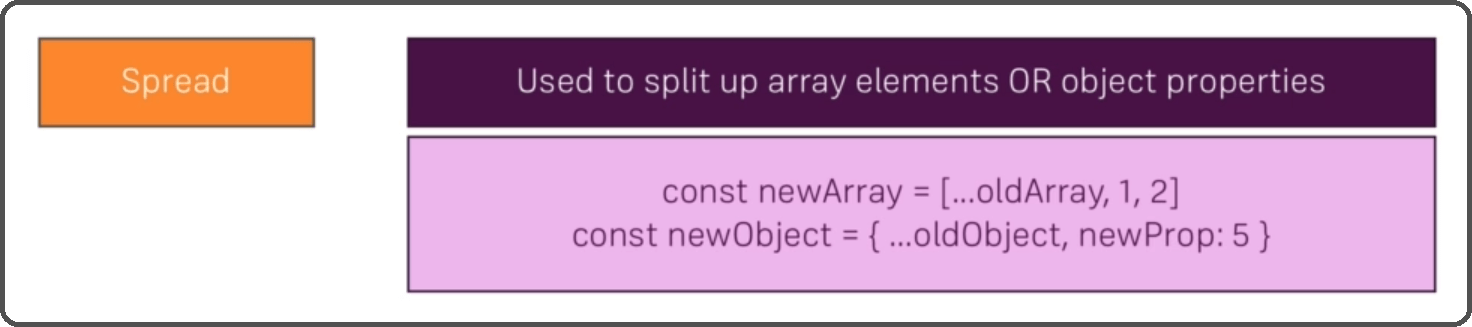The spread operator is used to split up array elements or object properties. So, we spread up an array or object. For example, if we have an old array and we want to add all the elements from that old array to a new array and additionally add the ‘1’ and ‘2’ elements. This first syntax would be what we use. Three dots in front of the old array will simply pull out all the elements and add them to the new array which we created with square brackets. And of course, then we can add more elements to it i.e. 1, 2. So if we just use the normal syntax with the square brackets to create an array.

The same for the object. We create a new object with curly braces with the newProp but then we also have three dots before the oldObject to pull out all the properties of the old object and their values and add them as key-value pairs to the new object. As a side note, if the old object also had a new property, it would be overwritten by a new Prop 5 here. So, our own property takes precedence. This is the spread operator in JavaScript.

##### Rest Operator in JavaScript:

Now the rest operator is the same operator but used differently. here it’s used to merge a list of function arguments into an array. And this shows us where we use it. We use it in a function argument list.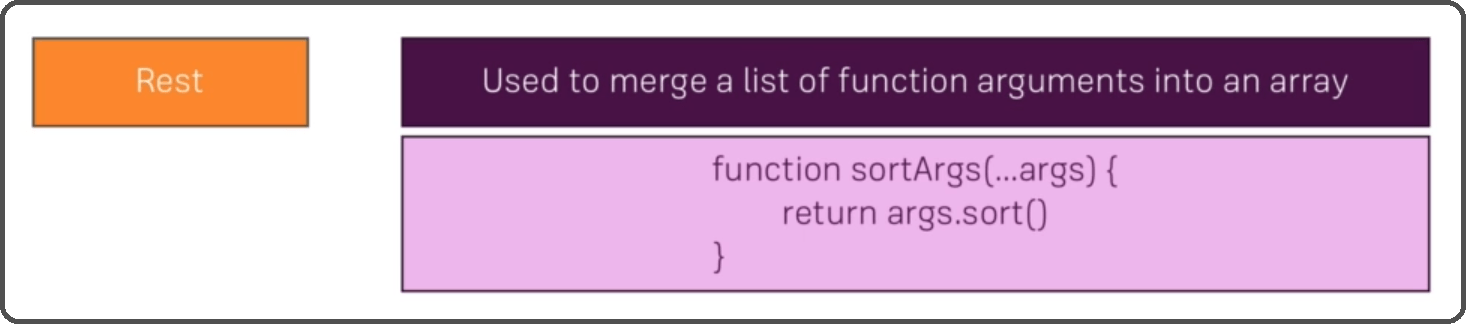For example, sortArgs receive an unlimited number of arguments. So, one argument, two, three, or whatever, with three dots, we only write one argument ‘args’ but we may actually receive more than one and they will all be merged together into an array. So, then we can apply array methods to our argument list or do whatever we want to do with our conveniently stored arguments. Let us have a look at both usages on JSBIN. So, let’s start with the spread operator.

```const numbers = [1, 2, 3];
const newNumbers = [...numbers, 4];
console.log(newNumbers);
```

Here, we have created an array of numbers i.e. 1,2,3. Add as many as you want. Then we created a new array newNumbers and as you saw spread is now simply used by adding three dots. Then the old array ‘numbers and then new numbers i.e., 4, and again that is optional. If we now console.log(newNumbers), the output will be,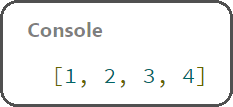It shows the old array with all its elements and the new element. If we remove the 3 dots before the numbers, it will display as one element inside the new element,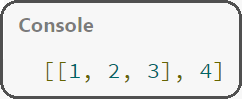So that’s the spread operator and you will see us use this throughout our React course a couple of times on both arrays and objects. For example, conveniently copy arrays or add properties to an object whilst safely copying that old object. We will obviously mention why we are using that operator. Let us now see the same for objects.

```const person = {
name: 'John'
}

const newPerson = {
...person,
age: 28
}

console.log(newPerson);
```

Here, we have created a person object where we have a name i.e. ‘John’. Then we have a newPerson object where first of all we have used the spread operator on person to copy all the property value pairs of the old object and that’s always optional. Then we have added a new property i.e., age: 32. And now if we console.log(newPerson),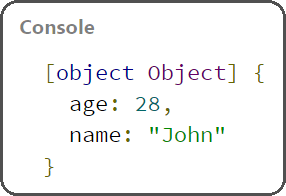You will see an object printed here with the name ‘John’ and age 32. So, it’s taking the old person and distributing it to the new person. That is the spread operator. Now the rest operator which is used less often though is used in a function and you could of course also use the ES6 arrow function.

```const filter = (...args) => {
return args.filter(el => el === 1);
}

console.log(filter(1,2,3));
```

So here, we have created a filter function (you can name it of your own choice) and there we have used the 3 dot operator or we can say we have a couple of args and you do not have to use args here. you can also name this of your own choice. But you have to use the three dots in front of it.

We could use the inline syntax here to write it all in one line without the return keyword here but we have used return. So then there you can simply return args.filter(), we have called the built-in filter method which is available on arrays, and keep in mind the dots here are used as a rest operator that merges the arguments into an array. So, we can use array methods like filters. The filter will execute a function on every element in the passed array.

So here, we have used the inline arrow function inside args.filter to simply say return true or false which filter expects if the element is equal to 1. Now, that might be a lot of syntaxes you don’t know. Here, three equals signs check for type and value equality so that el also has to be a number. And this is just an arrow function that we pass to the built-in filter method. So, this has nothing to do with rest or spread. Then we have console.log (filter (1, 2, 3)), the output will be 1 here as an array.Because we filtered this array which is created with the rest operator with the three dots here. So that’s Rest and Spread Operators in JavaScript.

In the next article, I am going to discuss Destructuring in JavaScript with Examples. Here, in this article, I try to explain Spread and Rest Operators in JavaScript with Examples. I hope you enjoy this Spread and Rest Operators in JavaScript article.